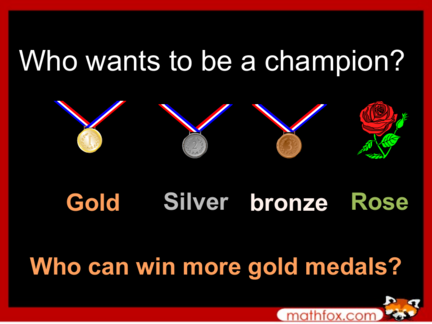Contributed by:1. Add 1 to 2 digit numbers.
2. Addition of 2 digit numbers.
5. Addition ways to make numbers.
1. Who wants to be a champion?
Gold Silver bronze Rose
Who can win more gold medals?
digit numbers numbers equations expression numbers
from pictures
3. Rose
10 + 3 =
4. Bronze medal
13 + 4 =
5. Silver medal
14 + 4 =
6. Gold medal
21 + 8 =
7. 10 + 10 =
8. Bronze medal
10 + 11 =
9. Silver medal
11 + 11 =
10. Gold medal
12 + 10 =
11. Rose
2 + __ = 1 + 3
12. Bronze medal
3 + 2 = __ + 6
13. Silver medal
9 = __ + 2 + 5
14. Gold medal
9 + 1 = 7 + __
15. Rose
Write the expression for the illustration.
16. Bronze medal
Write the expression for the illustration.
17. Silver medal
Write the expression for the illustration.
18. Gold medal
Write the expression for the illustration.
19. Rose
Say any addition expression that can make
the number below.
3
20. Bronze medal
Say any addition expression that can make
the number below.
7
21. Silver medal
Say any addition expression that can make
the number below.
9
22. Gold medal
Say any addition expression that can make
the number below.
10